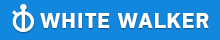GMAT Question of the Day - Daily to your Mailbox; hard ones only

 It is currently 26 Jun 2019, 03:44GMAT Club Daily Prep

Thank you for using the timer - this advanced tool can estimate your performance and suggest more practice questions. We have subscribed you to Daily Prep Questions via email.

Customized
for You

we will pick new questions that match your level based on your Timer History

Track

every week, we’ll send you an estimated GMAT score based on your performance

Practice
Pays

we will pick new questions that match your level based on your Timer History

Not interested in getting valuable practice questions and articles delivered to your email? No problem, unsubscribe here.Is 2^x > 75 ? (1) 3^x > 50 (2) 2^x*3^x > 1500

Author Message
TAGS:

Hide Tags

Math ExpertV
Joined: 02 Sep 2009
Posts: 55802
Is 2^x > 75 ? (1) 3^x > 50 (2) 2^x*3^x > 1500  [#permalink]

Show Tags00:00

Difficulty:55% (hard)

Question Stats:68% (02:19) correct32% (02:16) wrongbased on 58 sessions

HideShow timer StatisticsIs $$2^x > 75$$ ?

(1) $$3^x > 50$$

(2) $$2^x*3^x > 1500$$

_________________
examPAL RepresentativeP
Joined: 07 Dec 2017
Posts: 1072
Is 2^x > 75 ? (1) 3^x > 50 (2) 2^x*3^x > 1500  [#permalink]

Show Tags

Bunuel wrote:
Is $$2^x > 75$$ ?

(1) $$3^x > 50$$

(2) $$2^x*3^x > 1500$$

We should be familiar with the powers of 2 to know that 2^6 = 64 and 2^7 = 128.
So, (assuming x is an integer) we need to know if x > 6. Even if it isn't, this is a reasonable first approximation.

We'll look for statements that give us this information, a Logical approach.

(1) 3^3 = 27 and 3^4 = 81, so this tells us that x > 3. But is it larger than 6?
Insufficient.

(2) Again, we'll look for the edge of the range. We can either guess numbers or first use prime factorization: 1500 = 15 * 100 = 5*3*10^2 = 5*3*(5*2)^2 = (2^2)(3)(5^3). S

so if x = 6 then we can compare (2^6)(3^6) with (2^2)(3)(5^3). Canceling out (2^2)(3) from both gives (2^4)(3^5) = 16*243 on the left and 5^3 = 125 on the right.
So x = 6 is definitely enough. Since it can also be larger, this is once again insufficient.

Combining adds no new information (as all we have are inequalities and since x = 6 and x > 6 are good for both inequalities, they will be for their combination)

_________________
ManagerG
Joined: 10 Jan 2013
Posts: 231
Location: India
Concentration: General Management, Strategy
GPA: 3.95
Re: Is 2^x > 75 ? (1) 3^x > 50 (2) 2^x*3^x > 1500  [#permalink]

Show Tags

Can you please explaim stmt b
I am not clear post the prime factorization

Posted from my mobile device
examPAL RepresentativeP
Joined: 07 Dec 2017
Posts: 1072
Re: Is 2^x > 75 ? (1) 3^x > 50 (2) 2^x*3^x > 1500  [#permalink]

Show Tags

1
saurabh9gupta wrote:
Can you please explaim stmt b
I am not clear post the prime factorization

Posted from my mobile device

Hi,

Basically, if you plug x = 6 into statement (2) you get 2^x * 3^x = 2^6 * 3^6 which is larger than 1500. So, based on (2), x could equal 6 in which case 2^x < 75 and the answer is NO. But since x could also equal 7, and 2^7 is larger than 75 then this gives a YES. Two different answers - insufficient!

In the solution above, I used prime factorization because it gave me easier numbers to compare. Instead of actually calculating 2^6, 3^6 and multiplying, I could cancel out 4*3 = 12 from both 2^6*3^6 and 1500 which then let me make an easier calculation of 2^4 * 3^5. Doesn't really matter too much, just a question of how you can do the math as fast as possible. Directly approximating also works: 2^6 *3^6 = 64 * 27 * 27 = about 60 * 30 * 30 = much more than 1500, which is also perfectly fine.
_________________
ManagerS
Joined: 24 Dec 2017
Posts: 192
Location: India
Concentration: Strategy, Real Estate
Schools: Johnson '21
Re: Is 2^x > 75 ? (1) 3^x > 50 (2) 2^x*3^x > 1500  [#permalink]

Show Tags

2^x > 75?

We need to find the value of x to determine whether 2^x > 75. So what is the value of x?

St 1: 3^x > 50

3^1 = 3
3^2 = 9
3^3 = 27
3^4 = 81
3^5 = 243

In order to satisfy the statement, x should be greater than or equal to 4 (x>=4)
Now, lets apply this to the main question. 2^4 = 16, 2^5 = 32, 2^6 = 64, 2^7 = 128.
Here, 2^7 > 75 but 2^4 < 75. Hence the statement is insufficient .

St 2: 2^x ∗ 3^x > 1500

In order to satisfy this statement, x should be 5 or greater than 5. (2^5 ∗ 3^5 = 7776)
Back to the main question, 2^5 = 32. Is 32>75(No) but 2^7=128 which is greater than 75.
Therefore, the statement is clearly insufficient.

St 1+ St 2:

So far, we know from statement 1 and statement 2 that x>= 4 but that will not help us to get a unique answer. Therefore C is clearly insufficient too.

We are left with (E) & that's the answer IMO.

Thank you
ArjunRe: Is 2^x > 75 ? (1) 3^x > 50 (2) 2^x*3^x > 1500   [#permalink] 27 Nov 2018, 05:09
Display posts from previous: Sort by

Is 2^x > 75 ? (1) 3^x > 50 (2) 2^x*3^x > 1500